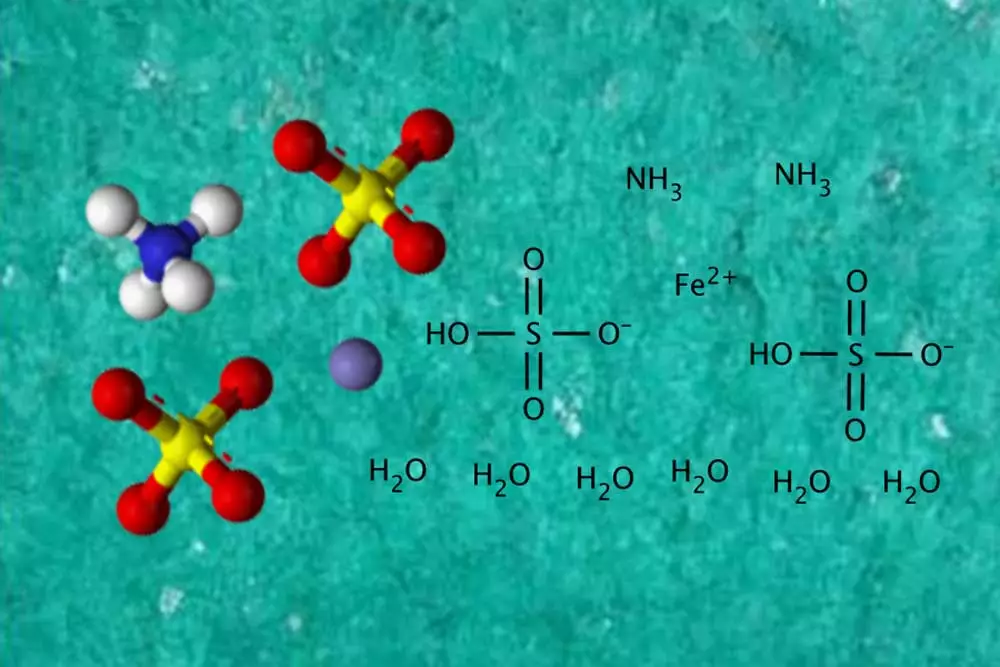// Important Properties and Applications of Ammonium Iron(II) Sulfate

# Important Properties and Applications of Ammonium Iron(II) Sulfate

Updated on January 11, 2021 | by adminAmmonium iron (II) sulfate is an inorganic chemical compound whose chemical formula can be written as (NH4)2Fe(SO4)2(H2O)6. Ammonium iron (II) sulfate is known to contain 2 different cations, namely the ammonium cation (denoted by the chemical formula NH4+) and the ferric cation (denoted by the chemical formula Fe2+). It also contains the sulfate anion (denoted by the chemical formula SO42-). This compound can, therefore, be classified as a double salt of ammonium sulfate and ferrous sulfate.

Ammonium iron (II) sulfate is a very common laboratory reagent that is commonly referred to as Mohr’s salt. Since it readily undergoes crystallization, this compound is relatively easy to store. Furthermore, it can be noted that the crystals of ammonium iron (II) sulfate are quite resistant towards oxidation in the presence of air. In a manner that is quite similar to other ferrous sulfate salts, ammonium iron (II) sulfate readily dissolves in water to give rise to an aquo complex with the formula [Fe(H2O)6]2+. It can be noted that this aquo complex is known to possess an octahedral molecular geometry. It can also be noted that the mineral form of ammonium iron (II) sulfate is commonly referred to as mohrite.

## Properties of Ammonium Iron (II) Sulfate

• The chemical formula of anhydrous ammonium iron (II) sulfate is Fe(SO4)(NH4)2(SO4). However, the chemical formula of the hexahydrate of this inorganic chemical compound must be represented as Fe(SO4)(NH4)2(SO4).6H2O.
• The molar mass of the anhydrous form of this inorganic compound is roughly equal to 284.05 grams per mole. The hexahydrate of Mohr’s salt is known to have a molar mass of roughly 392.13 grams per mole.
• Under standard conditions for temperature and pressure (often abbreviated to STP), the density of this compound is known to correspond to 1.86 grams per cubic centimetre.
• The melting point of ammonium iron (II) sulfate lies in the temperature range of 100 to 110 degrees Celsius and that makes it solid at room temperature.
• The hexahydrate form of this inorganic compound is known to be highly soluble in water. The solubility of the hexahydrate of Mohr’s salt in water corresponds to roughly 269 grams per litre.

## Applications

In the field of analytical chemistry, ammonium iron (II) sulfate is widely used as a source of ferrous ions. This compound is highly suitable for this application because it has a relatively long shelf life and is fairly resistant to oxidation. Note that oxidation involves the loss of electrons. For example, the olefin ethylene undergoes oxidation to yield ethylene oxide. Another vital application of ammonium iron (II) sulfate is for the measurement of high doses of gamma rays. This inorganic compound is used in Fricke’s dosimeter for this purpose. It can be noted that Mohr’s salt often contains impurities. Common impurities that may be present in ammonium iron (II) sulfate include manganese, magnesium, nickel, zinc, and lead. Many of these impurities are also known to form isomorphous salts.

Related Post